Blog

# 5 Ways To Get Students Excited About Forces And Free-body Diagrams

Grace Chukwuekwu
Teaching with Labster
October 31, 2022

When you push or pull on an object, you apply a force to that object. Force is an influence that can change the motion of an object. A force can cause an object with mass to change speed, i.e. in a hurry. A force has both a magnitude and a direction, making it a vector quantity.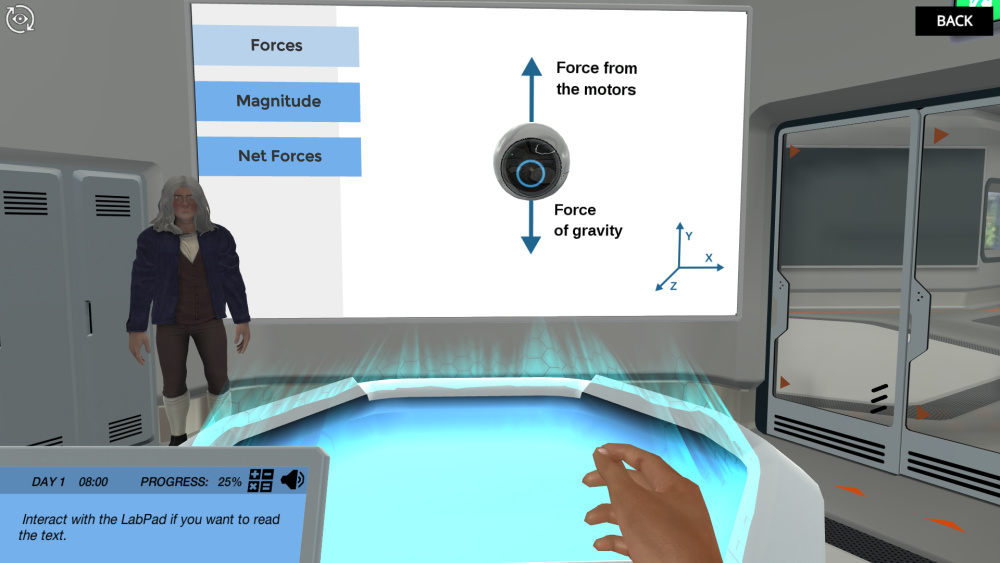Figure 1: An image extracted from the forces and free-body diagrams simulation from Labster. The simulation is useful for High School and University/College courses

Force is usually categorized by its source, transmission, or effect. These forces are generally responsible for the motion of objects as stated in Newton's laws of motion. External forces are all external forces acting on a body. A free-body diagram is a picture of all the external forces acting on the object, and when the forces on the system are balanced, the system is in equilibrium. The SI unit for force is the Newton (N), N = kg m/s2.

Read on for some thoughts on why this can be a worrying topic for teachers and students, five suggestions for improving the situation, and thoughts on why virtual labs can be useful.

## Why Can Force And Free-body Diagrams Be Complicated For Students?

There are three main reasons why forces and free-body diagrams can be difficult for even the most diligent student.

### 1. It feels abstract

Force occurs at a molecular level when two objects are in close proximity, you cannot see or feel it. Not being able to visualize the process and not seeing its bearing on the real world can discourage learning and make it difficult for students to stay motivated.

### 2. It's content-heavy

The normal force is the force exerted by a solid object to pass through it, also called normal force, because it acts perpendicular to the object's surface. It is usually denoted by N. An example of a normal force is a situation where an object stands on a table. The object's weight pulls it down through the mass, but the mass of the table exerts a normal upward force that offsets the object's weight.

Weight

The force acting on an object due to gravity is called its weight.

Friction

Friction is the resistive force that opposes the motion or tendency of an object to move.

Spring force

A spring is an object that will return to its original shape if it is deformed. To restore its shape, the spring exerts a force proportional to its displacement from its equilibrium position and in the direction opposite to that displacement. This is known as Hooke's Law.

Figure 2: The net force acting on a moving object (FG is the weight, fk is the kinetic friction, N is the normal force, and F is the pushing force)

### 3. It's complicated

The vector sum of all external forces acting on an object or system is called the net external force Fnet and is also represented as F. According to Newton's second law of motion, the net force causes an acceleration on an acting object as long as its value is not zero. For example, consider the case where an object is pulled up by a buoyant force and pulled down by its weight. The net force acting on this object is the vector sum of the buoyant force and the weight.

Since the net force is a vector sum, calculating the net force often involves adding vectors. Often (when the force is moving in more than one direction), the easiest way to do this is to factor the vector along two different axes of the coordinate system and then add the components along each axis separately. A useful tool that can help with this is the free-body diagrams.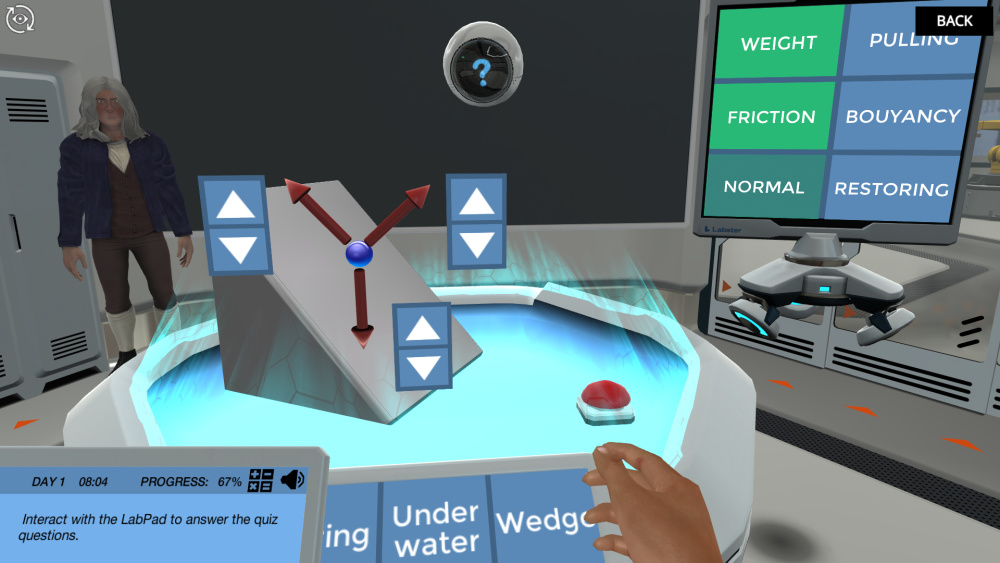Labster's forces and free-body diagrams simulation.

## 5 Ways To Make Forces And Free-body Diagrams More Accessible Topics

With these points in mind, here are five things you can incorporate into the Force and Free-Body Diagram lesson to make teaching more engaging, accessible, and enjoyable for you and your students.

### 1. Show the people behind the science

Sir Isaac Newton described the motion of all bodies in terms of momentum and force, discovering that they obeyed certain laws of conservation. In 1687 Newton published his dissertation Philosophiæ Naturalis Principia Mathematica. In this work, Newton described three laws of motion that are still the way forces are explained in physics today.

### 2. Relate to the real world

Force can be divided into two categories;

1. Contact force arises from direct physical contact between objects. For example, the restoring force of a spring on an attached mass.

2. Field forces operate without requiring physical contact between objects and depend on the existence of a "field" in the region of space surrounding the object in question. Since the mass is in the earth's gravitational field, it feels the force of gravity; in other words, it has weight.

### 3. Seeing is believing

When an object moves, it often experiences resistance because the object interacts with its environment. Imagine the case of sliding down a sand dune as opposed to an iceberg. The resistance you experience from sand is friction.

Friction opposes the relative motion between systems in contact. Friction is a common but complex force. The direction of friction is always opposite to the direction of motion, parallel to the surface between the objects and perpendicular to the normal force. An object can experience static and kinetic friction.

The center of mass is the average position of all the parts of the system weighed by their masses. Take an apple as an example. An apple has many parts, leaves, skin, seeds, etc. Consider the weight of all these parts and try to draw their distance from a point in the middle. This point is a unique position where the weighted position vectors of all parts of the system add up to zero. The position of the center of mass has units of meters. It is not necessary to have the actual object's matter at the center of mass. Consider the case of a balloon where the center of mass is in the center where there is only air.

Center of gravity

In equilibrium situations, one of the factors influencing a body is its weight. In a free-body diagram, the weight vector depends on the object's center of gravity. The center of gravity and the center of mass can be at different points and only if the object is so large that the gravitational field is not uniform throughout its volume. In practice, however, the center of gravity is identical to the center of mass.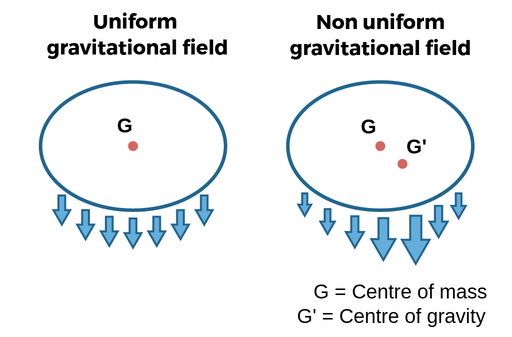Figure 6: Mass and center of gravity in uniform and non-uniform fields.

### 4. Provide enough details

A free-body diagram is a picture of all the external forces acting on an object. It is a useful tool for analyzing many problems related to force and motion. Complete the following steps to create a free-body diagram: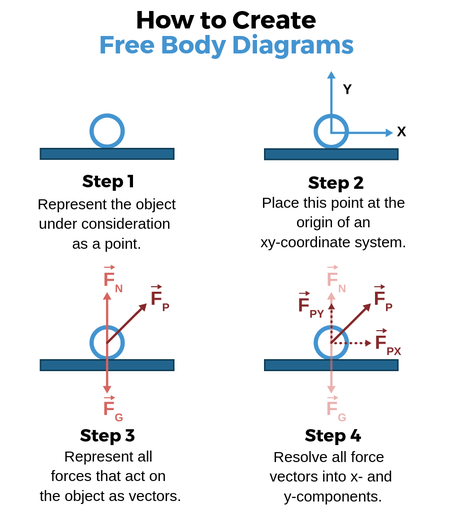Figure 7: Steps to create a free-body diagram.

Optional Step: If the problem contains two or more objects, draw a separate free-body diagram for each object.

In most cases, it is not necessary to represent every force vector with exact magnitude and direction (especially since this is sometimes unknown). In contrast, free-body diagrams are most useful as a qualitative technique for organizing information that will be used to perform quantitative calculations later.

### 5. Encourage the use of virtual laboratory simulation

A unique way to teach forces and free-body diagrams is through virtual laboratory simulations. At Labster, we are dedicated to providing fully interactive state-of-the-art lab simulations that use gamified elements such as storytelling and scoring systems in an immersive, 3D world.

Check out the Labster forces and free-body diagrams simulation that allows students to learn about forces and free-body diagrams through active, inquiry-based learning. In the simulation, students will team up with Sir Isaac Newton and understand how various forces affect the motion of objects.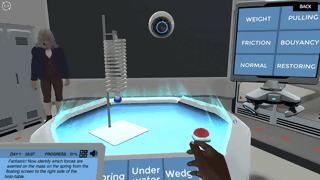Learn more about the forces and free-body diagrams simulation here or get in touch to find out how you can start using virtual labs with your students.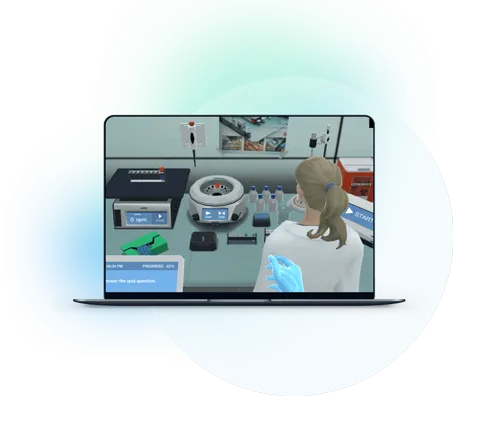What exactly are virtual labs?